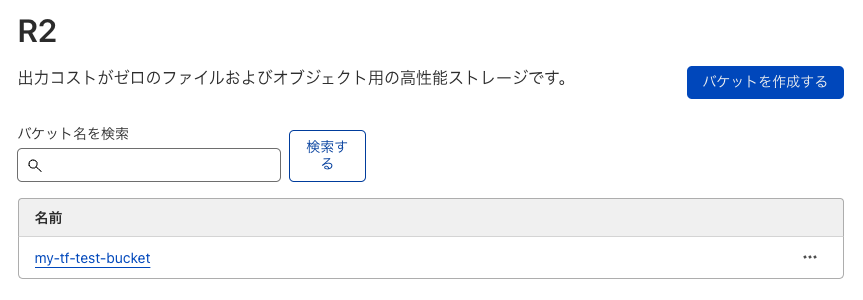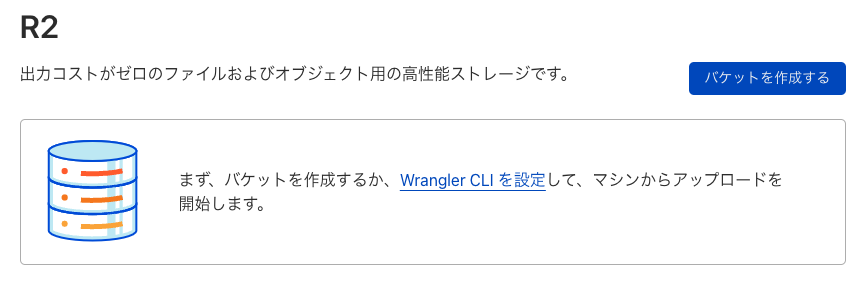# Terraform で Cloudflare R2 のバケットを作成する

2022/10/08

Terraform の肩慣らしとして、Cloudflare R2 のバケットを作成してみた

Cloudflare R2 のドキュメントに書いてあったものを参考にした
https://developers.cloudflare.com/r2/examples/terraform/

## Terraform バージョン

``````\$ terraform -v
Terraform v1.3.2
on darwin_amd64``````

## 手順

### 設定ファイルを作成

main.tf
``````terraform {
required_providers {
aws = {
source = "hashicorp/aws"
version = "4.34.0"
}
}
}

provider "aws" {
access_key = "access key"
secret_key = "secret key"
skip_credentials_validation = true
skip_region_validation = true
skip_requesting_account_id = true
endpoints {
s3 = "https://<account id>.r2.cloudflarestorage.com"
}
region = "auto"
}

resource "aws_s3_bucket" "cloudflare-bucket" {
bucket = "my-tf-test-bucket"
}``````

access key と secret key は R2 の管理画面、「R2 API トークンの管理」から作成できる

account id は R2 の管理画面の右上あたりに表示されている

region はドキュメントに書いていなかったが、実行した時に以下のエラーが出たので追記した

``Error: creating Amazon S3 (Simple Storage) Bucket (my-tf-test-bucket): InvalidRegionName: The region name 'ap-northeast-1' is not valid. Must be 'auto'``

### ワークスペースの初期化

``\$ terraform init``

### 実行計画の確認

``\$ terraform plan``

### 実行

``\$ terraform apply``

``````Do you want to perform these actions?
Terraform will perform the actions described above.
Only 'yes' will be accepted to approve.

Enter a value: yes``````

``````\$ tree .
.
├── main.tf
├── terraform.tfstate
└── terraform.tfstate.backup``````

R2 の管理画面でバケットが作成されていることが確認できた### 片づけ

``\$ terraform destroy``

バケットが消えていることを確認## 実行ログ

バケットを作成するだけだが、たくさん項目が出てきてちょっと不安になった

### terraform init

``````\$ terraform init

Initializing the backend...

Initializing provider plugins...
- Finding hashicorp/aws versions matching "4.34.0"...
- Installing hashicorp/aws v4.34.0...
- Installed hashicorp/aws v4.34.0 (signed by HashiCorp)

Terraform has created a lock file .terraform.lock.hcl to record the provider
selections it made above. Include this file in your version control repository
so that Terraform can guarantee to make the same selections by default when
you run "terraform init" in the future.

Terraform has been successfully initialized!

You may now begin working with Terraform. Try running "terraform plan" to see
any changes that are required for your infrastructure. All Terraform commands
should now work.

If you ever set or change modules or backend configuration for Terraform,
rerun this command to reinitialize your working directory. If you forget, other
commands will detect it and remind you to do so if necessary.``````

### terraform plan

``````\$ terraform plan

Terraform used the selected providers to generate the following execution plan. Resource actions are indicated with the following symbols:
+ create

Terraform will perform the following actions:

# aws_s3_bucket.cloudflare-bucket will be created
+ resource "aws_s3_bucket" "cloudflare-bucket" {
+ acceleration_status         = (known after apply)
+ acl                         = (known after apply)
+ arn                         = (known after apply)
+ bucket                      = "my-tf-test-bucket"
+ bucket_domain_name          = (known after apply)
+ bucket_regional_domain_name = (known after apply)
+ force_destroy               = false
+ hosted_zone_id              = (known after apply)
+ id                          = (known after apply)
+ object_lock_enabled         = (known after apply)
+ policy                      = (known after apply)
+ region                      = (known after apply)
+ request_payer               = (known after apply)
+ tags_all                    = (known after apply)
+ website_domain              = (known after apply)
+ website_endpoint            = (known after apply)

+ cors_rule {
+ allowed_headers = (known after apply)
+ allowed_methods = (known after apply)
+ allowed_origins = (known after apply)
+ expose_headers  = (known after apply)
+ max_age_seconds = (known after apply)
}

+ grant {
+ id          = (known after apply)
+ permissions = (known after apply)
+ type        = (known after apply)
+ uri         = (known after apply)
}

+ lifecycle_rule {
+ abort_incomplete_multipart_upload_days = (known after apply)
+ enabled                                = (known after apply)
+ id                                     = (known after apply)
+ prefix                                 = (known after apply)
+ tags                                   = (known after apply)

+ expiration {
+ date                         = (known after apply)
+ days                         = (known after apply)
+ expired_object_delete_marker = (known after apply)
}

+ noncurrent_version_expiration {
+ days = (known after apply)
}

+ noncurrent_version_transition {
+ days          = (known after apply)
+ storage_class = (known after apply)
}

+ transition {
+ date          = (known after apply)
+ days          = (known after apply)
+ storage_class = (known after apply)
}
}

+ logging {
+ target_bucket = (known after apply)
+ target_prefix = (known after apply)
}

bject_lock_configuration {
+ object_lock_enabled = (known after apply)

+ rule {
+ default_retention {
+ days  = (known after apply)
+ mode  = (known after apply)
+ years = (known after apply)
}
}
}

+ replication_configuration {
+ role = (known after apply)

+ rules {
+ delete_marker_replication_status = (known after apply)
+ id                               = (known after apply)
+ prefix                           = (known after apply)
+ priority                         = (known after apply)
+ status                           = (known after apply)

+ destination {
+ account_id         = (known after apply)
+ bucket             = (known after apply)
+ replica_kms_key_id = (known after apply)
+ storage_class      = (known after apply)

+ access_control_translation {
+ owner = (known after apply)
}

+ metrics {
+ minutes = (known after apply)
+ status  = (known after apply)
}

+ replication_time {
+ minutes = (known after apply)
+ status  = (known after apply)
}
}

+ filter {
+ prefix = (known after apply)
+ tags   = (known after apply)
}

+ source_selection_criteria {
+ sse_kms_encrypted_objects {
+ enabled = (known after apply)
}
}
}
}

+ server_side_encryption_configuration {
+ rule {
+ bucket_key_enabled = (known after apply)

+ apply_server_side_encryption_by_default {
+ kms_master_key_id = (known after apply)
+ sse_algorithm     = (known after apply)
}
}
}

+ versioning {
+ enabled    = (known after apply)
+ mfa_delete = (known after apply)
}

+ website {
+ error_document           = (known after apply)
+ index_document           = (known after apply)
+ redirect_all_requests_to = (known after apply)
+ routing_rules            = (known after apply)
}
}

Plan: 1 to add, 0 to change, 0 to destroy.

─────────────────────────────────────────────────────────────────────────────────────────────────────────────────────────────────────────────────────

Note: You didn't use the -out option to save this plan, so Terraform can't guarantee to take exactly these actions if you run "terraform apply" now.``````

### terraform apply

``````\$ terraform apply

Terraform used the selected providers to generate the following execution plan. Resource actions are indicated with the following symbols:
+ create

Terraform will perform the following actions:

# aws_s3_bucket.cloudflare-bucket will be created
+ resource "aws_s3_bucket" "cloudflare-bucket" {
+ acceleration_status         = (known after apply)
+ acl                         = (known after apply)
+ arn                         = (known after apply)
+ bucket                      = "my-tf-test-bucket"
+ bucket_domain_name          = (known after apply)
+ bucket_regional_domain_name = (known after apply)
+ force_destroy               = false
+ hosted_zone_id              = (known after apply)
+ id                          = (known after apply)
+ object_lock_enabled         = (known after apply)
+ policy                      = (known after apply)
+ region                      = (known after apply)
+ request_payer               = (known after apply)
+ tags_all                    = (known after apply)
+ website_domain              = (known after apply)
+ website_endpoint            = (known after apply)

+ cors_rule {
+ allowed_headers = (known after apply)
+ allowed_methods = (known after apply)
+ allowed_origins = (known after apply)
+ expose_headers  = (known after apply)
+ max_age_seconds = (known after apply)
}

+ grant {
+ id          = (known after apply)
+ permissions = (known after apply)
+ type        = (known after apply)
+ uri         = (known after apply)
}

+ lifecycle_rule {
+ abort_incomplete_multipart_upload_days = (known after apply)
+ enabled                                = (known after apply)
+ id                                     = (known after apply)
+ prefix                                 = (known after apply)
+ tags                                   = (known after apply)

+ expiration {
+ date                         = (known after apply)
+ days                         = (known after apply)
+ expired_object_delete_marker = (known after apply)
}

+ noncurrent_version_expiration {
+ days = (known after apply)
}

+ noncurrent_version_transition {
+ days          = (known after apply)
+ storage_class = (known after apply)
}

+ transition {
+ date          = (known after apply)
+ days          = (known after apply)
+ storage_class = (known after apply)
}
}

+ logging {
+ target_bucket = (known after apply)
+ target_prefix = (known after apply)
}

+ object_lock_configuration {
+ object_lock_enabled = (known after apply)

+ rule {
+ default_retention {
+ days  = (known after apply)
+ mode  = (known after apply)
+ years = (known after apply)
}
}
}

+ replication_configuration {
+ role = (known after apply)

+ rules {
+ delete_marker_replication_status = (known after apply)
+ id                               = (known after apply)
+ prefix                           = (known after apply)
+ priority                         = (known after apply)
+ status                           = (known after apply)

+ destination {
+ account_id         = (known after apply)
+ bucket             = (known after apply)
+ replica_kms_key_id = (known after apply)
+ storage_class      = (known after apply)

+ access_control_translation {
+ owner = (known after apply)
}

+ metrics {
+ minutes = (known after apply)
+ status  = (known after apply)
}

+ replication_time {
+ minutes = (known after apply)
+ status  = (known after apply)
}
}

+ filter {
+ prefix = (known after apply)
+ tags   = (known after apply)
}

+ source_selection_criteria {
+ sse_kms_encrypted_objects {
+ enabled = (known after apply)
}
}
}
}

+ server_side_encryption_configuration {
+ rule {
+ bucket_key_enabled = (known after apply)

+ apply_server_side_encryption_by_default {
+ kms_master_key_id = (known after apply)
+ sse_algorithm     = (known after apply)
}
}
}

+ versioning {
+ enabled    = (known after apply)
+ mfa_delete = (known after apply)
}

+ website {
+ error_document           = (known after apply)
+ index_document           = (known after apply)
+ redirect_all_requests_to = (known after apply)
+ routing_rules            = (known after apply)
}
}

Plan: 1 to add, 0 to change, 0 to destroy.

Do you want to perform these actions?
Terraform will perform the actions described above.
Only 'yes' will be accepted to approve.

Enter a value: yes

aws_s3_bucket.cloudflare-bucket: Creating...
aws_s3_bucket.cloudflare-bucket: Creation complete after 4s [id=my-tf-test-bucket]

Apply complete! Resources: 1 added, 0 changed, 0 destroyed.``````

### terraform destroy

``````\$ terraform destroy
aws_s3_bucket.cloudflare-bucket: Refreshing state... [id=my-tf-test-bucket]

Terraform used the selected providers to generate the following execution plan. Resource actions are indicated with the following symbols:
- destroy

Terraform will perform the following actions:

# aws_s3_bucket.cloudflare-bucket will be destroyed
- resource "aws_s3_bucket" "cloudflare-bucket" {
- arn                         = "arn::s3:::my-tf-test-bucket" -> null
- bucket                      = "my-tf-test-bucket" -> null
- bucket_domain_name          = "my-tf-test-bucket.s3.amazonaws.com" -> null
- bucket_regional_domain_name = "my-tf-test-bucket.s3.amazonaws.com" -> null
- force_destroy               = false -> null
- hosted_zone_id              = "<Masked Value>" -> null
- id                          = "my-tf-test-bucket" -> null
- object_lock_enabled         = false -> null
- region                      = "us-east-1" -> null
- tags                        = {} -> null
- tags_all                    = {} -> null

- grant {
- id          = "<Masked Value>" -> null
- permissions = [
- "FULL_CONTROL",
] -> null
- type        = "CanonicalUser" -> null
}

- lifecycle_rule {
- abort_incomplete_multipart_upload_days = 7 -> null
- enabled                                = false -> null
- tags                                   = {} -> null
}

- server_side_encryption_configuration {
- rule {
- bucket_key_enabled = true -> null

- apply_server_side_encryption_by_default {
- sse_algorithm = "AES256" -> null
}
}
}

- versioning {
- enabled    = false -> null
- mfa_delete = false -> null
}
}

Plan: 0 to add, 0 to change, 1 to destroy.

Do you really want to destroy all resources?
Terraform will destroy all your managed infrastructure, as shown above.
There is no undo. Only 'yes' will be accepted to confirm.

Enter a value: yes

aws_s3_bucket.cloudflare-bucket: Destroying... [id=my-tf-test-bucket]
aws_s3_bucket.cloudflare-bucket: Destruction complete after 1s

Destroy complete! Resources: 1 destroyed.``````